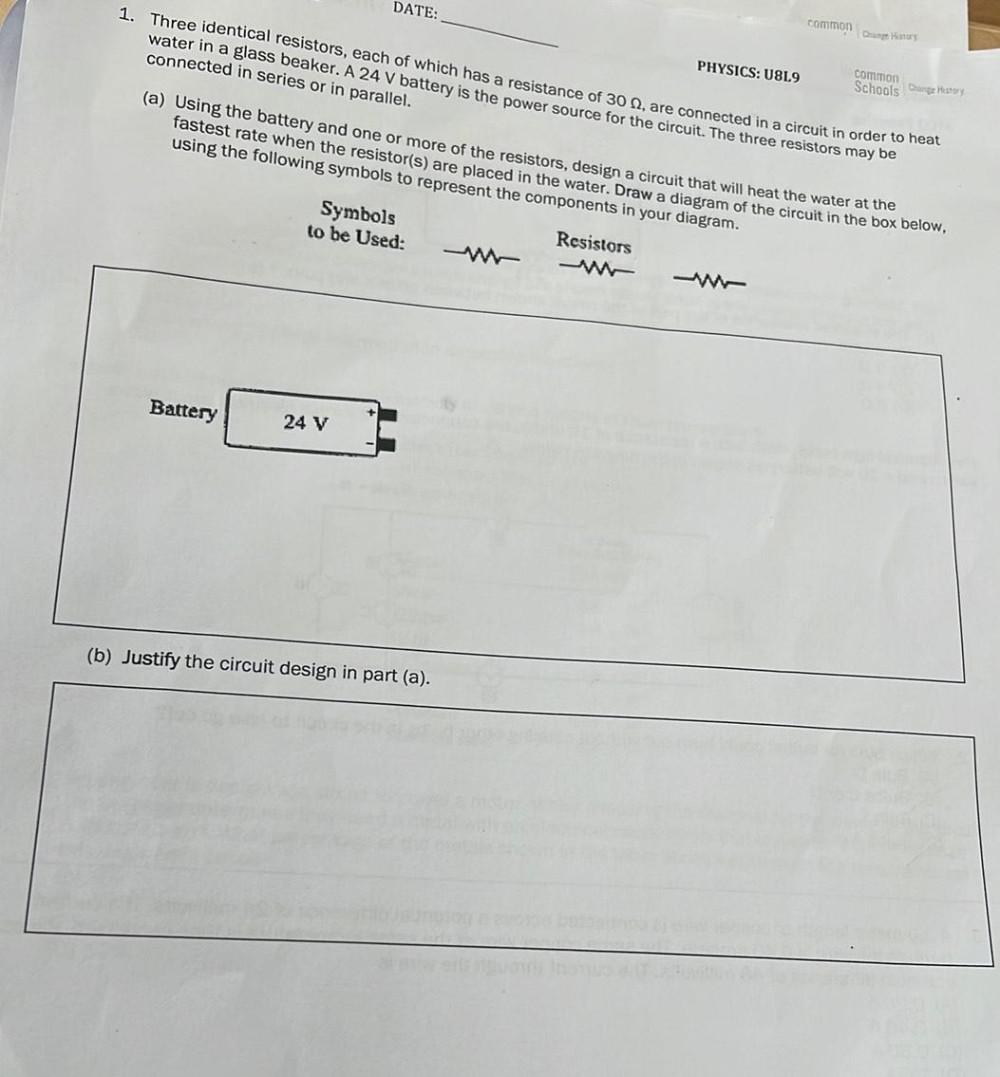Question:

# Three identical resistors, each of which has a resistance of 30 Ω, are connected in a circuit in order to heat water in a glass beaker. A 24 V battery is the power source for the circuit . The three rThree identical resistors, each of which has a resistance of 30 Ω, are connected in a circuit in order to heat water in a glass beaker. A 24 V battery is the power source for the circuit . The three resistors may be connected in series or in parallel. (a) Using the battery and one or more of the resistors, design a circuit that will heat the water at the fastest rate when the resistor(s) are placed in the water. Draw a diagram of the circuit in the box below, using the following symbols to represent the components in your diagram. Symbols to be Used : Resistors (b) Justify the circuit design in part (a).